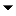﻿ Operations > Strategy Analyzer > Optimization > Optimization Fitness Metrics

Optimization Fitness Metrics

 Navigation:  Operations > Strategy Analyzer > Optimization > Optimization Fitness MetricsShow/Hide Hidden Text

Optimization fitness metrics are used as the targets of optimization tests to determine the optimal mix of strategy parameter values. Below is a list of all pre-loaded optimization fitness metrics and their definitions. Custom optimization fitness metrics can be developed via NinjaScript, as well.Understanding Max % ProfitableUnderstanding Max Avg. Favorable ExcursionUnderstanding Max Avg. ProfitUnderstanding Max Net ProfitUnderstanding Max Profit FactorUnderstanding Max R Squared (R^2)Understanding Max Sharpe RatioUnderstanding Max Sortino RatioUnderstanding Max Ulcer RatioUnderstanding Max Win/Loss RatioUnderstanding Minimum Avg. Adverse ExcursionUnderstanding Min DrawdownUnderstanding Max Strength